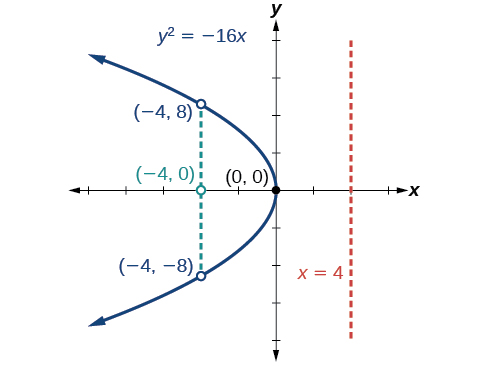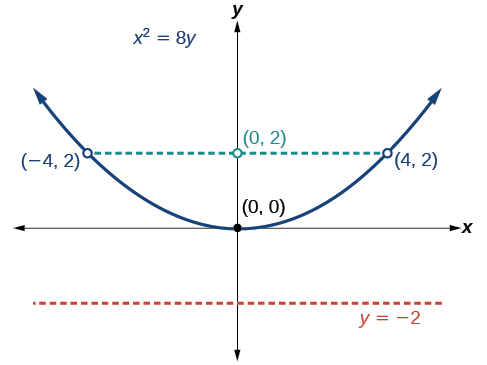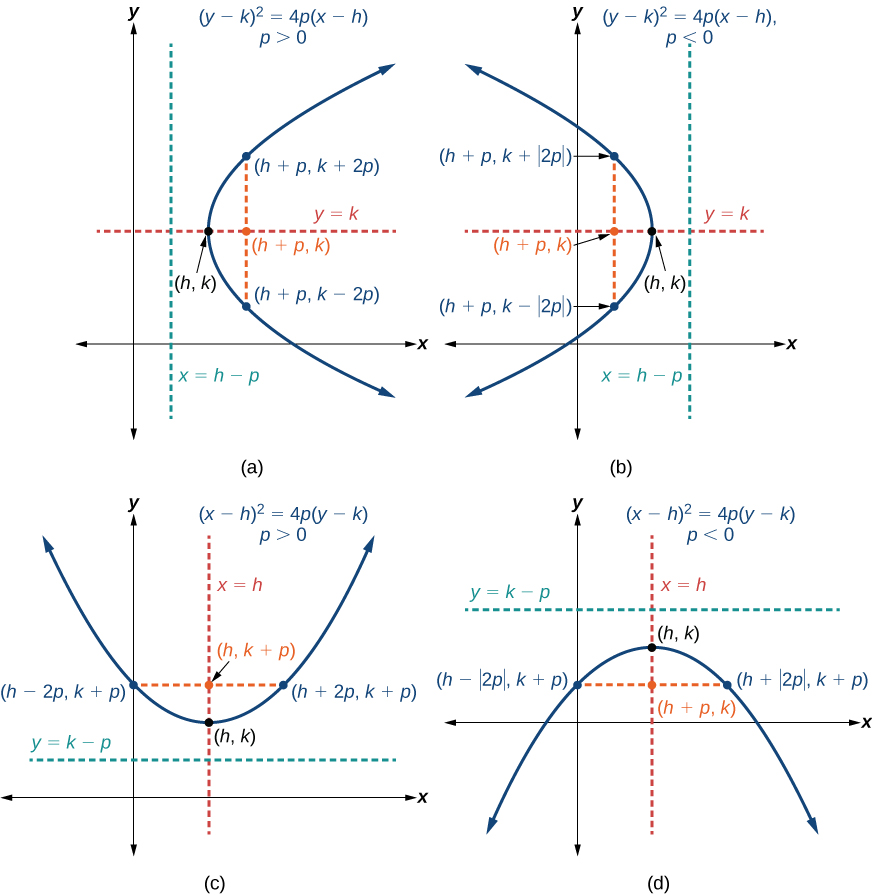# 12.3 The parabola  (Page 3/11)

 Page 3 / 11

Graph $\text{\hspace{0.17em}}{y}^{2}=-16x.\text{\hspace{0.17em}}$ Identify and label the focus, directrix, and endpoints of the latus rectum.

Focus: $\text{\hspace{0.17em}}\left(-4,0\right);\text{\hspace{0.17em}}$ Directrix: $\text{\hspace{0.17em}}x=4;\text{\hspace{0.17em}}$ Endpoints of the latus rectum: $\text{\hspace{0.17em}}\left(-4,±8\right)$## Graphing a parabola with vertex (0, 0) and the y -axis as the axis of symmetry

Graph $\text{\hspace{0.17em}}{x}^{2}=-6y.\text{\hspace{0.17em}}$ Identify and label the focus , directrix    , and endpoints of the latus rectum    .

The standard form that applies to the given equation is $\text{\hspace{0.17em}}{x}^{2}=4py.\text{\hspace{0.17em}}$ Thus, the axis of symmetry is the y -axis. It follows that:

• $-6=4p,$ so $\text{\hspace{0.17em}}p=-\frac{3}{2}.\text{\hspace{0.17em}}$ Since $\text{\hspace{0.17em}}p<0,$ the parabola opens down.
• the coordinates of the focus are $\text{\hspace{0.17em}}\left(0,p\right)=\left(0,-\frac{3}{2}\right)$
• the equation of the directrix is $\text{\hspace{0.17em}}y=-p=\frac{3}{2}$
• the endpoints of the latus rectum can be found by substituting into the original equation, $\text{\hspace{0.17em}}\left(±3,-\frac{3}{2}\right)$

Next we plot the focus, directrix, and latus rectum, and draw a smooth curve to form the parabola    .

Graph $\text{\hspace{0.17em}}{x}^{2}=8y.\text{\hspace{0.17em}}$ Identify and label the focus, directrix, and endpoints of the latus rectum.

Focus: $\text{\hspace{0.17em}}\left(0,2\right);\text{\hspace{0.17em}}$ Directrix: $\text{\hspace{0.17em}}y=-2;\text{\hspace{0.17em}}$ Endpoints of the latus rectum: $\text{\hspace{0.17em}}\left(±4,2\right).$## Writing equations of parabolas in standard form

In the previous examples, we used the standard form equation of a parabola to calculate the locations of its key features. We can also use the calculations in reverse to write an equation for a parabola when given its key features.

Given its focus and directrix, write the equation for a parabola in standard form.

1. Determine whether the axis of symmetry is the x - or y -axis.
1. If the given coordinates of the focus have the form $\text{\hspace{0.17em}}\left(p,0\right),$ then the axis of symmetry is the x -axis. Use the standard form $\text{\hspace{0.17em}}{y}^{2}=4px.$
2. If the given coordinates of the focus have the form $\text{\hspace{0.17em}}\left(0,p\right),$ then the axis of symmetry is the y -axis. Use the standard form $\text{\hspace{0.17em}}{x}^{2}=4py.$
2. Multiply $\text{\hspace{0.17em}}4p.$
3. Substitute the value from Step 2 into the equation determined in Step 1.

## Writing the equation of a parabola in standard form given its focus and directrix

What is the equation for the parabola    with focus $\text{\hspace{0.17em}}\left(-\frac{1}{2},0\right)\text{\hspace{0.17em}}$ and directrix     $\text{\hspace{0.17em}}x=\frac{1}{2}?$

The focus has the form $\text{\hspace{0.17em}}\left(p,0\right),$ so the equation will have the form $\text{\hspace{0.17em}}{y}^{2}=4px.$

• Multiplying $\text{\hspace{0.17em}}4p,$ we have $\text{\hspace{0.17em}}4p=4\left(-\frac{1}{2}\right)=-2.$
• Substituting for $\text{\hspace{0.17em}}4p,$ we have $\text{\hspace{0.17em}}{y}^{2}=4px=-2x.$

Therefore, the equation for the parabola is $\text{\hspace{0.17em}}{y}^{2}=-2x.$

What is the equation for the parabola with focus $\text{\hspace{0.17em}}\left(0,\frac{7}{2}\right)\text{\hspace{0.17em}}$ and directrix $\text{\hspace{0.17em}}y=-\frac{7}{2}?$

${x}^{2}=14y.$

## Graphing parabolas with vertices not at the origin

Like other graphs we’ve worked with, the graph of a parabola can be translated. If a parabola is translated $\text{\hspace{0.17em}}h\text{\hspace{0.17em}}$ units horizontally and $\text{\hspace{0.17em}}k\text{\hspace{0.17em}}$ units vertically, the vertex will be $\text{\hspace{0.17em}}\left(h,k\right).\text{\hspace{0.17em}}$ This translation results in the standard form of the equation we saw previously with $\text{\hspace{0.17em}}x\text{\hspace{0.17em}}$ replaced by $\text{\hspace{0.17em}}\left(x-h\right)\text{\hspace{0.17em}}$ and $\text{\hspace{0.17em}}y\text{\hspace{0.17em}}$ replaced by $\text{\hspace{0.17em}}\left(y-k\right).$

To graph parabolas with a vertex $\text{\hspace{0.17em}}\left(h,k\right)\text{\hspace{0.17em}}$ other than the origin, we use the standard form $\text{\hspace{0.17em}}{\left(y-k\right)}^{2}=4p\left(x-h\right)\text{\hspace{0.17em}}$ for parabolas that have an axis of symmetry parallel to the x -axis, and $\text{\hspace{0.17em}}{\left(x-h\right)}^{2}=4p\left(y-k\right)\text{\hspace{0.17em}}$ for parabolas that have an axis of symmetry parallel to the y -axis. These standard forms are given below, along with their general graphs and key features.

## Standard forms of parabolas with vertex ( h , k )

[link] and [link] summarize the standard features of parabolas with a vertex at a point $\text{\hspace{0.17em}}\left(h,k\right).$

 Axis of Symmetry Equation Focus Directrix Endpoints of Latus Rectum $y=k$ ${\left(y-k\right)}^{2}=4p\left(x-h\right)$ $x=h-p$ $x=h$ ${\left(x-h\right)}^{2}=4p\left(y-k\right)$ $y=k-p$(a) When   p > 0 , the parabola opens right. (b) When   p < 0 , the parabola opens left. (c) When   p > 0 , the parabola opens up. (d) When   p < 0 , the parabola opens down.

given that x= 3/5 find sin 3x
4
DB
remove any signs and collect terms of -2(8a-3b-c)
-16a+6b+2c
Will
Joeval
(x2-2x+8)-4(x2-3x+5)
sorry
Miranda
x²-2x+9-4x²+12x-20 -3x²+10x+11
Miranda
x²-2x+9-4x²+12x-20 -3x²+10x+11
Miranda
(X2-2X+8)-4(X2-3X+5)=0 ?
master
The anwser is imaginary number if you want to know The anwser of the expression you must arrange The expression and use quadratic formula To find the answer
master
The anwser is imaginary number if you want to know The anwser of the expression you must arrange The expression and use quadratic formula To find the answer
master
Y
master
master
Soo sorry (5±Root11* i)/3
master
Mukhtar
explain and give four example of hyperbolic function
What is the correct rational algebraic expression of the given "a fraction whose denominator is 10 more than the numerator y?
y/y+10
Mr
Find nth derivative of eax sin (bx + c).
Find area common to the parabola y2 = 4ax and x2 = 4ay.
Anurag
A rectangular garden is 25ft wide. if its area is 1125ft, what is the length of the garden
to find the length I divide the area by the wide wich means 1125ft/25ft=45
Miranda
thanks
Jhovie
What do you call a relation where each element in the domain is related to only one value in the range by some rules?
A banana.
Yaona
given 4cot thither +3=0and 0°<thither <180° use a sketch to determine the value of the following a)cos thither
what are you up to?
nothing up todat yet
Miranda
hi
jai
hello
jai
Miranda Drice
jai
aap konsi country se ho
jai
which language is that
Miranda
I am living in india
jai
good
Miranda
what is the formula for calculating algebraic
I think the formula for calculating algebraic is the statement of the equality of two expression stimulate by a set of addition, multiplication, soustraction, division, raising to a power and extraction of Root. U believe by having those in the equation you will be in measure to calculate it
Miranda
state and prove Cayley hamilton therom
hello
Propessor
hi
Miranda
the Cayley hamilton Theorem state if A is a square matrix and if f(x) is its characterics polynomial then f(x)=0 in another ways evey square matrix is a root of its chatacteristics polynomial.
Miranda
hi
jai
hi Miranda
jai
thanks
Propessor
welcome
jai
What is algebra
algebra is a branch of the mathematics to calculate expressions follow.
Miranda
Miranda Drice would you mind teaching me mathematics? I think you are really good at math. I'm not good at it. In fact I hate it. 😅😅😅
Jeffrey
lolll who told you I'm good at it
Miranda
something seems to wispher me to my ear that u are good at it. lol
Jeffrey
lolllll if you say so
Miranda
but seriously, Im really bad at math. And I hate it. But you see, I downloaded this app two months ago hoping to master it.
Jeffrey
which grade are you in though
Miranda
oh woww I understand
Miranda
Jeffrey
Jeffrey
Miranda
how come you finished in college and you don't like math though
Miranda
gotta practice, holmie
Steve
if you never use it you won't be able to appreciate it
Steve
I don't know why. But Im trying to like it.
Jeffrey
yes steve. you're right
Jeffrey
so you better
Miranda
what is the solution of the given equation?
which equation
Miranda
I dont know. lol
Jeffrey
Miranda
Jeffrey
answer and questions in exercise 11.2 sums
how do u calculate inequality of irrational number?
Alaba
give me an example
Chris
and I will walk you through it
Chris
cos (-z)= cos z .
cos(- z)=cos z
MustafaByByBy OpenStaxBy OpenStaxBy OpenStaxBy Robert MurphyBy RhodesBy OpenStaxBy John GabrieliBy David CoreyBy Jill ZerressenBy Richley Crapo